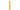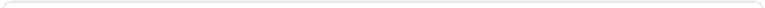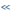Sign InContactsupport: Customer Portal Focused on delivering choice, investment protection and flexibility to organizations with valuable COBOL assets

Veryant Knowledge Base
Home > All Categories > isCOBOL General > Where can I find more information on A\$ENCRYPT and A\$DECRYPT?Where can I find more information on A\$ENCRYPT and A\$DECRYPT?

Question:

Where can I find more information on A\$ENCRYPT and A\$DECRYPT? I am getting errors involving 'padding' and 'multiple of 8 bytes' when I use these routines.

The size of the key passed to A\$ENCRYPT or A\$DECRYPT must be less than or equal to 128-bits (i.e. 16 bytes). For example, use the following data item for your encryption key: ``` 77 encryption-key pic x(16). ``` When A\$ENCRYPT returns, the length of the encrypted-data is set to an exact value, and in A\$DECRYPT the length of data-to-decrypt must be exact. Use items defined as PIC X ANY LENGTH to ensure that you can retrieve and set the lengths precisely. For example, use the following data items for your encrypted-data and data-to-decrypt: ``` 77 encrypted-data pic x any length. 77 data-to-decrypt pic x any length. ```

Then, for example, you can take the output from A\$ENCRYPT (i.e. encrypted-data) and pass it as the input to A\$DECRYPT (i.e. data-to-decrypt) to reverse the encryption.

See the attached sample program, encryption.cbl

Note that the encrypted data is binary and is not an encoded character string.

The A\$ENCRYPT routine does the equivalent of the following Java code: ``` public final static String CRYPT_ALGORITHM = "Blowfish"; Cipher cipher = Cipher.getInstance(CRYPT_ALGORITHM); cipher.init(Cipher.ENCRYPT_MODE, new SecretKeySpec(encryptionKey, 0, encryptionKey.length, CRYPT_ALGORITHM)); byte[] encryptedData = cipher.doFinal(dataToEncrypt, 0, dataToEncrypt.length)); ``` where dataToEncrypt, encryptionKey and encryptedData are the 3 parameters passed to A\$ENCRYPT.

The A\$DECRYPT routine does the equivalent of the following Java code:

``` public final static String CRYPT_ALGORITHM = "Blowfish"; Cipher cipher = Cipher.getInstance(CRYPT_ALGORITHM); cipher.init(Cipher.DECRYPT_MODE, new SecretKeySpec(encryptionKey, 0, encryptionKey.length, CRYPT_ALGORITHM)); byte[] decryptedData = cipher.doFinal(dataToDecrypt, 0, dataToDecrypt.length)); ``` where dataToDecrypt, encryptionKey and decryptedData are the 3 parameters passed to A\$DECRYPT.

Detailed information can be found in the Java Cryptography Architecture (JCA) Reference Guide here.

Authored by: Veryant Support This question has been viewed 10744 times so far.File AttachmentsUser CommentsRelated QuestionsAdditional Information Article Number: 138 Created: 2010-07-21 12:12 PM Rating:Article OptionsPrint this QuestionEmail Question to FriendExport to PDF FileExport to MS WordSubscribe to ArticleSearch Knowledge BaseKnowledge Base Home